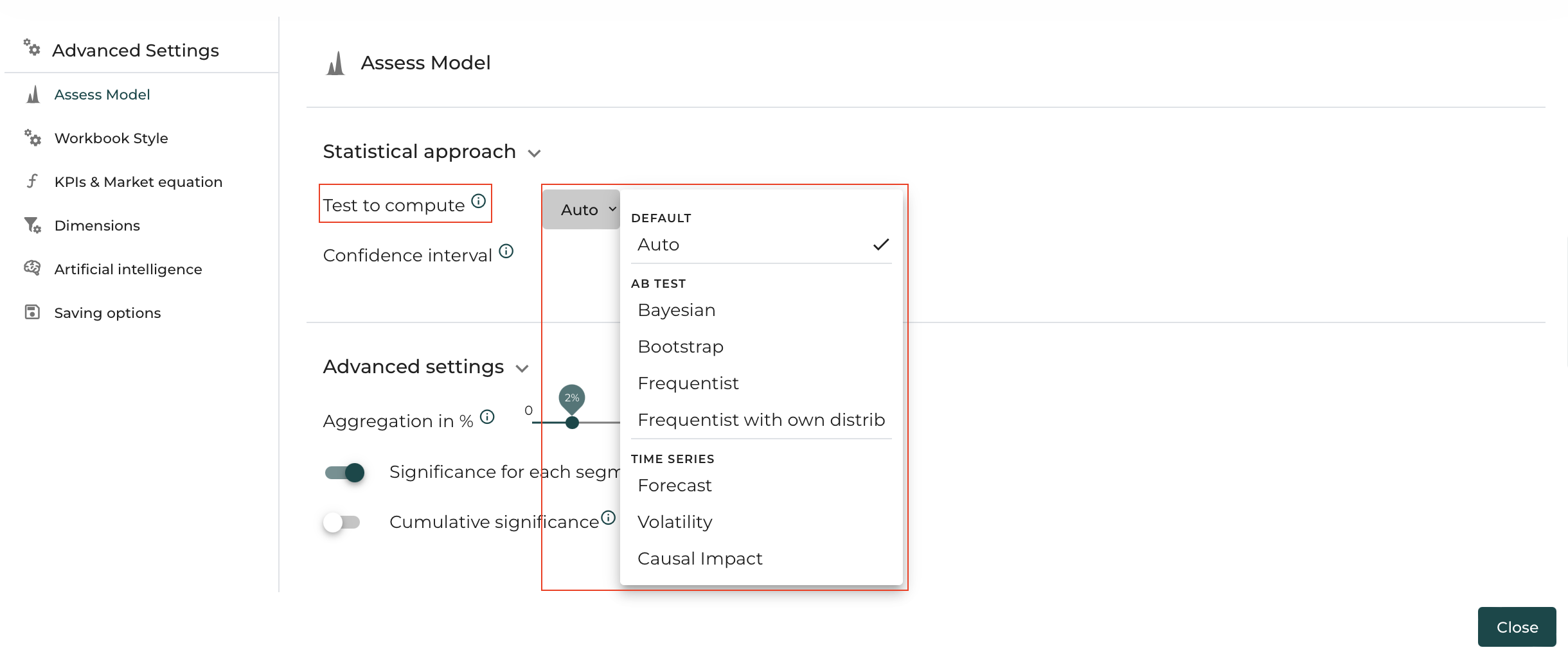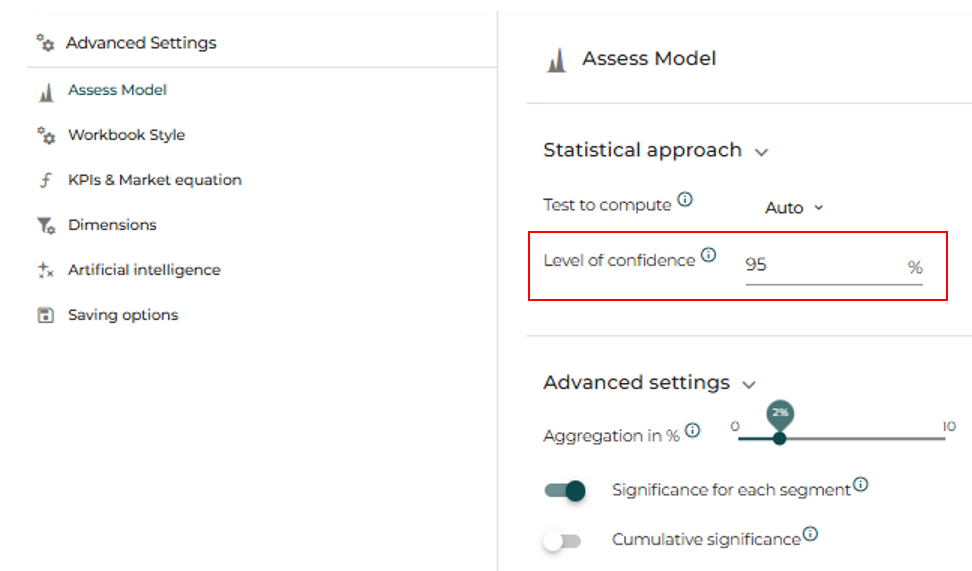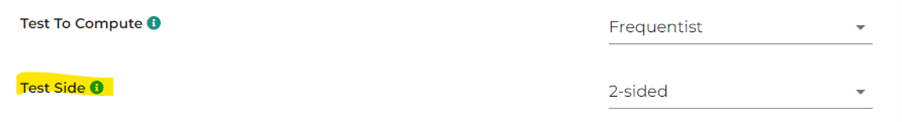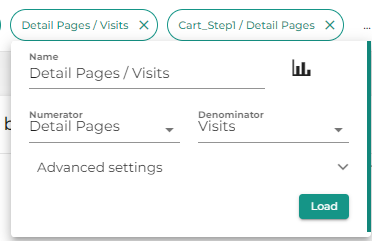# Assess - Settings

Here is the different settings available in DataMa Assess

Here’s what you going to find in this chapter:

Statistical approach:

Basic settings:

# Statistical approach

## Test to Compute

You can decide which test to perform depending by selecting the appropriate model. Please refer to the Model section for more details## Confidence interval

Confidence interval is set in % to decide the level of confidence, or statistical power of your assessment. It ranges from 50% to 99%.

Depending on the test you are performing, this input can be used in different ways. Please refer to the Model section for more details## Frequentist Weight

The Frequentist Weight parameter allows you to select a metric that will multiply the number of occurrences of a given line by that metric.

For instance, if you’re analyzing the significance of a variation of Average Basket value in an AB test, you would need to have 1 line per transaction, so that DataMa can compute the standard deviation of that Revenues generated per transaction. However, since you have many transactions, you could end up with a too large dataset. To avoid handling large datasets, DataMa allows you to define a “weight” for each line, and in that example you could group transactions by bucket of Revenues. This dataset is a good example of that transformation from this other dataset, where “Lines” will be used as denominator for the KPI and “Weight” column as the weightening for Revenue/ Lines ratio. Note that the second data set is significantly smaller than the first one, and gives the same results.

## Impacted Dimension

Causal Impact test allows to assess the significance of a variation pre-post an intervention on a test group. To read more about the Causal Impact

Define the dimension impacted by the change, that were you have you “Control” and “Variant”.

## Impacted Segment

Causal Impact test allows to assess the significance of a variation pre-post an intervention on a test group. To read more about the Causal Impact

Define which is the “Variant” segment within the Dimension selected above. This segment is the one impacted by the change

## Impact Sizing

Impact Sizing tells you how important is the relationship between two segments in a specific metric.

e.g. Significance between “Test A” and “Test B” can be sized in Revenue or in Purchase, or marge…

## Test side

In the Settings, you can define to compute the test results with one-sided or two-sided approach for frequentist and bayesian tests.Long story short, for a typical AB test, 1-sided test readouts allows you to be confident that B is higher than A, and not that B is lower than A

In Statistics hypothesis testing, we need to judge whether it is a one-tailed or a two-tailed test so that we can find the critical values in tables such as Standard Normal z Distribution Table and t Distribution Table. And then, by comparing test statistic value with the critical value or whether the statistical value falls in the critical region, we make a conclusion either to reject the null hypothesis or to fail to reject the null hypothesis.

How can we tell whether it is a one-tailed or a two-tailed test? It depends on the original claim in the question. A one-tailed test looks for an “increase” or “decrease” in the parameter whereas a two-tailed test looks for a “change” (could be increase or decrease) in the parameter. Therefore, if we see words such as “increased, greater, larger, improved and so on”, or “decreased, less, smaller and so on” in the original claim of a question (>,’<’are used in H1), a one-tail test is applied. If words such as “change, the same, different/difference and so on” are used in the claim of the question (≠is used in H1), a two-tailed test is applied.

## Aggregation in %

Level of aggregation: The level of aggregation that the model is using

e.g. if Level of aggregation is set at X%, segment within each dimension that represents less than X% of the Primary Numerator (e.g. Revenues) of the main KPI you’re analyzing will be clustered in one « Other » segment. X is set at 2 by default, but you may want to play with this parameter quite a bit because it can change significantly the calculation of mix effects.

• Click on the drop-down arrow to display the settings menu

• Move the cursor to the right to increase the level of aggregation

• Segments are now aggregated at the requested level

## Significance for each Segment

This parameter is activated by default. This allows to calculate the significance for every Dimension, and for every Segment within Dimensions. Deactivate this parameter to accelerate time of calculation.

## Cumulative significance

This parameter is de-activated by default. Instead of calculating the significance independantly for every segment in a dimension, it will add every segment with the previous one. This is interesting when you are mesuring the significance for date, it will allows you to measure at which date you reached the significance.

# Basic settings

## Comparison

First thing you need to do in DataMa Assess is to define the comparison on which you want to assess the significance.

Please refer to the Dimension Comparison section

## KPI

In DataMa Assess, you can assess the significance of a variation only on one given KPI. Hence, you need to select one of the step of your metric relation for that KPI. By default, DataMa selects the first ratio of your metric relation, but you can change that by clicking on the KPI bar in the subheader and ticking the appropriate metric within your metric relationIf you want to assess the significance for a KPI that is not explicitly in your metric relation, you can still edit the numerator and denominator manually by clicking on the KPI pill. See specific section for more details on the KPI pill